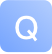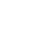# 将分数和权重分配给线段-问答-阿里云开发者社区-阿里云## 将分数和权重分配给线段

origin, age, amount, etc

def age(value):

``````if value < 3:
Score = 1
elif 3 <= value < 5:
Score = 7
elif value >= 5:
Score = 10
else:
Score = 10
Weight = 5
return (Score, Weight)
``````

def origin(value):

``````if value.upper() == "USA":
Score = 1
elif value.upper() == "JAPAN":
Score = 1
elif value.upper() == "CHINA":
Score = 10
elif value.upper() == "OTHER":
Score = 7
else:
Score = 10
Weight = 1
return (Score, Weight)``````

[[(7, 1)], [(1, 1)]]...

## calling all 40 functions doesnt seem efficient
lst.append([age(4)])
lst.append([origin("JAPAN")])

score = item[0 for item in lst]
weight = item[0 for item in lst]
scoresum = (listsum(score))
weightsum = (listsum(weight))

total = scoresum/weightsum

1 条回答写回答取消 提交回答
• 如果你坚持总结元组，你可以通过使用这个技巧简化该操作：

# Option 1

[sum(x) for x in zip(*lst)]

# Option 2

map(sum, zip(*l))
这将产生一个总和列表（例如[25, 32]），然后您可以使用它来计算总和。

但是，您需要有一个扁平的元组列表，这是您可能想要进行的更改。该列表应如下所示：

[(2, 1), (1, 1), ...]

2019-07-17 23:26:09
赞同 展开评论 打赏

434
1
0
126
1
0
123
1
0
91
1
0
69
1
0
170
1
0
82
1
0
69
1
0
968
2
0
179
1
0